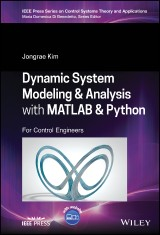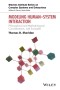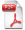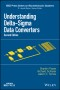# Details## Dynamic System Modelling and Analysis with MATLAB and Python

For Control Engineers
IEEE Press Series on Control Systems Theory and Applications 1. Aufl.
 von: Jongrae Kim 103,99 € Verlag: Wiley Format: PDF Veröffentl.: 26.09.2022 ISBN/EAN: 9781119801634 Sprache: englisch Anzahl Seiten: 336

DRM-geschütztes eBook, Sie benötigen z.B. Adobe Digital Editions und eine Adobe ID zum Lesen.

## Beschreibungen

#### Titelbeschreibung

<b>Dynamic System Modeling & Analysis with MATLAB & Python</b> <p><b>A robust introduction to the advanced programming techniques and skills needed for control engineering </b> <p>In <i>Dynamic System Modeling & Analysis with MATLAB & Python: For Control Engineers</i>, accomplished control engineer Dr. Jongrae Kim delivers an insightful and concise introduction to the advanced programming skills required by control engineers. The book discusses dynamic systems used by satellites, aircraft, autonomous robots, and biomolecular networks. Throughout the text, MATLAB and Python are used to consider various dynamic modeling theories and examples. <p> The author covers a range of control topics, including attitude dynamics, attitude kinematics, autonomous vehicles, systems biology, optimal estimation, robustness analysis, and stochastic system. An accompanying website includes a solutions manual as well as MATLAB and Python example code. <p><i>Dynamic System Modeling & Analysis with MATLAB & Python: For Control Engineers</i> provides readers with a sound starting point to learning programming in the engineering or biology domains. It also offers: <ul><li>A thorough introduction to attitude estimation and control, including attitude kinematics and sensors and extended Kalman filters for attitude estimation</li> <li>Practical discussions of autonomous vehicles mission planning, including unmanned aerial vehicle path planning and moving target tracking</li> <li>Comprehensive explorations of biological network modeling, including bio-molecular networks and stochastic modeling</li> <li>In-depth examinations of control algorithms using biomolecular networks, including implementation</li></ul> <p><i>Dynamic System Modeling & Analysis with MATLAB & Python: For Control Engineers</i> is an indispensable resource for advanced undergraduate and graduate students seeking practical programming instruction for dynamic system modeling and analysis using control theory.

#### Inhaltsverzeichnis

<p>Preface xiii</p> <p>Acknowledgements xv</p> <p>Acronyms xvii</p> <p>About the Companion Website xix</p> <p>1 Introduction 1</p> <p>1.1 Scope of the Book 1</p> <p>1.2 Motivation Examples 2</p> <p>1.2.1 Free-Falling Object 2</p> <p>1.2.1.1 First Program in Matlab 4</p> <p>1.2.1.2 First Program in Python 10</p> <p>1.2.2 Ligand–Receptor Interactions 14</p> <p>1.3 Organization of the Book 21</p> <p>Exercises 21</p> <p>Bibliography 22</p> <p>2 Attitude Estimation and Control 23</p> <p>2.1 Attitude Kinematics and Sensors 23</p> <p>2.1.1 Solve Quaternion Kinematics 26</p> <p>2.1.1.1 MATLAB 26</p> <p>2.1.1.2 Python 29</p> <p>2.1.2 Gyroscope Sensor Model 33</p> <p>2.1.2.1 Zero-Mean Gaussian White Noise 33</p> <p>2.1.2.2 Generate Random Numbers 34</p> <p>2.1.2.3 Stochastic Process 40</p> <p>2.1.2.4 MATLAB 41</p> <p>2.1.2.5 Python 45</p> <p>2.1.2.6 Gyroscope White Noise 49</p> <p>2.1.2.7 Gyroscope RandomWalk Noise 50</p> <p>2.1.2.8 Gyroscope Simulation 53</p> <p>2.1.3 Optical Sensor Model 57</p> <p>2.2 Attitude Estimation Algorithm 64</p> <p>2.2.1 A Simple Algorithm 64</p> <p>2.2.2 QUEST Algorithm 65</p> <p>2.2.3 Kalman Filter 66</p> <p>2.2.4 Extended Kalman Filter 75</p> <p>2.2.4.1 Error Dynamics 76</p> <p>2.2.4.2 Bias Noise 77</p> <p>2.2.4.3 Noise Propagation in Error Dynamics 78</p> <p>2.2.4.4 State Transition Matrix, Φ 84</p> <p>2.2.4.5 Vector Measurements 84</p> <p>2.2.4.6 Summary 86</p> <p>2.2.4.7 Kalman Filter Update 86</p> <p>2.2.4.8 Kalman Filter Propagation 87</p> <p>2.3 Attitude Dynamics and Control 88</p> <p>2.3.1 Dynamics Equation of Motion 88</p> <p>2.3.1.1 MATLAB 91</p> <p>2.3.1.2 Python 94</p> <p>2.3.2 Actuator and Control Algorithm 95</p> <p>2.3.2.1 MATLAB Program 98</p> <p>2.3.2.2 Python 101</p> <p>2.3.2.3 Attitude Control Algorithm 103</p> <p>2.3.2.4 Altitude Control Algorithm 105</p> <p>2.3.2.5 Simulation 106</p> <p>2.3.2.6 MATLAB 107</p> <p>2.3.2.7 Robustness Analysis 107</p> <p>2.3.2.8 Parallel Processing 110</p> <p>Exercises 113</p> <p>Bibliography 115</p> <p>3 Autonomous Vehicle Mission Planning 119</p> <p>3.1 Path Planning 119</p> <p>3.1.1 Potential Field Method 119</p> <p>3.1.1.1 MATLAB 122</p> <p>3.1.1.2 Python 126</p> <p>3.1.2 Graph Theory-Based Sampling Method 126</p> <p>3.1.2.1 MATLAB 128</p> <p>3.1.2.2 Python 129</p> <p>3.1.2.3 Dijkstra’s Shortest Path Algorithm 130</p> <p>3.1.2.4 MATLAB 130</p> <p>3.1.2.5 Python 131</p> <p>3.1.3 Complex Obstacles 134</p> <p>3.1.3.1 MATLAB 135</p> <p>3.1.3.2 Python 141</p> <p>3.2 Moving Target Tracking 145</p> <p>3.2.1 UAV and Moving Target Model 145</p> <p>3.2.2 Optimal Target Tracking Problem 148</p> <p>3.2.2.1 MATLAB 149</p> <p>3.2.2.2 Python 151</p> <p>3.2.2.3 Worst-Case Scenario 153</p> <p>3.2.2.4 MATLAB 157</p> <p>3.2.2.5 Python 159</p> <p>3.2.2.6 Optimal Control Input 164</p> <p>3.3 Tracking Algorithm Implementation 167</p> <p>3.3.1 Constraints 167</p> <p>3.3.1.1 Minimum Turn Radius Constraints 167</p> <p>3.3.1.2 Velocity Constraints 169</p> <p>3.3.2 Optimal Solution 172</p> <p>3.3.2.1 Control Input Sampling 172</p> <p>3.3.2.2 Inside the Constraints 175</p> <p>3.3.2.3 Optimal Input 177</p> <p>3.3.3 Verification Simulation 180</p> <p>Exercises 182</p> <p>Bibliography 182</p> <p>4 Biological System Modelling 185</p> <p>4.1 Biomolecular Interactions 185</p> <p>4.2 Deterministic Modelling 185</p> <p>4.2.1 Group of Cells and Multiple Experiments 186</p> <p>4.2.1.1 Model Fitting and the Measurements 188</p> <p>4.2.1.2 Finding Adaptive Parameters 190</p> <p>4.2.2 E. coli Tryptophan Regulation Model 191</p> <p>4.2.2.1 Steady-State and Dependant Parameters 194</p> <p>4.2.2.2 Padé Approximation of Time-Delay 195</p> <p>4.2.2.3 State-Space Realization 196</p> <p>4.2.2.4 Python 205</p> <p>4.2.2.5 Model Parameter Ranges 206</p> <p>4.2.2.6 Model Fitting Optimization 213</p> <p>4.2.2.7 Optimal Solution (MATLAB) 221</p> <p>4.2.2.8 Optimal Solution (Python) 223</p> <p>4.2.2.9 Adaptive Parameters 226</p> <p>4.2.2.10 Limitations 226</p> <p>4.3 Biological Oscillation 227</p> <p>4.3.1 Gillespie’s Direct Method 231</p> <p>4.3.2 Simulation Implementation 234</p> <p>4.3.3 Robustness Analysis 241</p> <p>Exercises 245</p> <p>Bibliography 246</p> <p>5 Biological System Control 251</p> <p>5.1 Control Algorithm Implementation 251</p> <p>5.1.1 PI Controller 251</p> <p>5.1.1.1 Integral Term 252</p> <p>5.1.1.2 Proportional Term 253</p> <p>5.1.1.3 Summation of the Proportional and the Integral Terms 253</p> <p>5.1.1.4 Approximated PI Controller 253</p> <p>5.1.1.5 Comparison of PI Controller and the Approximation 254</p> <p>5.1.2 Error Calculation: ΔP 260</p> <p>5.2 Robustness Analysis: 𝜇-Analysis 269</p> <p>5.2.1 Simple Examples 269</p> <p>5.2.1.1 𝜇 Upper Bound 272</p> <p>5.2.1.2 𝜇 Lower Bound 275</p> <p>5.2.1.3 Complex Numbers in MATLAB/Python 278</p> <p>5.2.2 Synthetic Circuits 280</p> <p>5.2.2.1 MATLAB 281</p> <p>5.2.2.2 Python 281</p> <p>5.2.2.3 𝜇-Upper Bound: Geometric Approach 290</p> <p>Exercises 291</p> <p>Bibliography 292</p> <p>6 FurtherReadings295</p> <p>6.1 Boolean Network 295</p> <p>6.2 Network Structure Analysis 296</p> <p>6.3 Spatial-Temporal Dynamics 297</p> <p>6.4 Deep Learning Neural Network 298</p> <p>6.5 Reinforcement Learning 298</p> <p>Bibliography 298</p> <p>Appendix A Solutions for Selected Exercises 301</p> <p>A.1 Chapter 1 301</p> <p>Exercise 1.4 301</p> <p>Exercise 1.5 301</p> <p>A.2 Chapter 2 302</p> <p>Exercise 2.5 302</p> <p>A.3 Chapter 3 302</p> <p>Exercise 3.1 302</p> <p>Exercise 3.6 303</p> <p>A.4 Chapter 4 303</p> <p>Exercise 4.1 303</p> <p>Exercise 4.2 303</p> <p>Exercise 4.7 304</p> <p>A.5 Chapter 5 304</p> <p>Exercise 5.2 304</p> <p>Exercise 5.3 304</p> <p>Index 307</p>

#### Autorenportrait

<p><b>Jongrae Kim, PhD,</b> is Associate Professor at the Institute of Design, Robotics & Optimization (iDRO), School of Mechanical Engineering, University of Leeds in the United Kingdom. He obtained his doctorate from the Department of Aerospace Engineering at Texas A&M University in the United States in 2002. </p>

#### Back cover copy

<p><b>A robust introduction to the advanced programming techniques and skills needed for control engineering </b> </p> <p>In <i>Dynamic System Modeling & Analysis with MATLAB & Python: For Control Engineers</i>, accomplished control engineer Dr. Jongrae Kim delivers an insightful and concise introduction to the advanced programming skills required by control engineers. The book discusses dynamic systems used by satellites, aircraft, autonomous robots, and biomolecular networks. Throughout the text, MATLAB and Python are used to consider various dynamic modeling theories and examples. <p> The author covers a range of control topics, including attitude dynamics, attitude kinematics, autonomous vehicles, systems biology, optimal estimation, robustness analysis, and stochastic system. An accompanying website includes a solutions manual as well as MATLAB and Python example code. <p><i>Dynamic System Modeling & Analysis with MATLAB & Python: For Control Engineers</i> provides readers with a sound starting point to learning programming in the engineering or biology domains. It also offers: <ul><li>A thorough introduction to attitude estimation and control, including attitude kinematics and sensors and extended Kalman filters for attitude estimation</li> <li>Practical discussions of autonomous vehicles mission planning, including unmanned aerial vehicle path planning and moving target tracking</li> <li>Comprehensive explorations of biological network modeling, including bio-molecular networks and stochastic modeling</li> <li>In-depth examinations of control algorithms using biomolecular networks, including implementation</li></ul> <p><i>Dynamic System Modeling & Analysis with MATLAB & Python: For Control Engineers</i> is an indispensable resource for advanced undergraduate and graduate students seeking practical programming instruction for dynamic system modeling and analysis using control theory.

US

## Diese Produkte könnten Sie auch interessieren:Modeling HumanSystem Interaction
von: Thomas B. Sheridan97,99 €Understanding Delta-Sigma Data Converters
von: Shanthi Pavan, Richard Schreier, Gabor C. Temes122,99 €